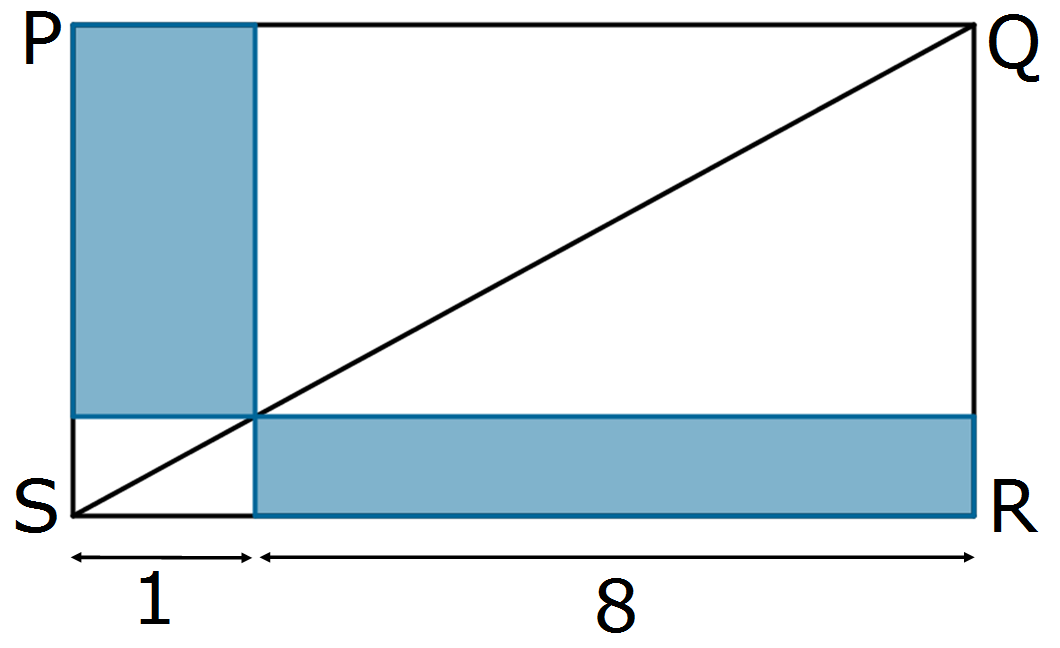#### You may also like### Rationals Between...

What fractions can you find between the square roots of 65 and 67?### F'arc'tion

At the corner of the cube circular arcs are drawn and the area enclosed shaded. What fraction of the surface area of the cube is shaded? Try working out the answer without recourse to pencil and paper.### Tiny Nines

What do you notice about these families of recurring decimals?

# Diagonal Touch

##### Age 14 to 16 Short Challenge Level:What fraction of rectangle $PQRS$ is shaded?

If you liked this problem, here is an NRICH task which challenges you to use similar mathematical ideas.
This problem is taken from the UKMT Mathematical Challenges.
You can find more short problems, arranged by curriculum topic, in our short problems collection.